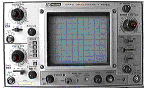## Hands-On-Physics: SOUND - Extension #3

### SPEED OF SOUND

There are many methods for measuring the speed of sound. The method outlined below uses the phenomenon of wave interference to determine the speed of sound.

This extension involves measurements of sound intensity in a closed space. You will need learn about the superpostion of waves and build experimental apparatus for investigating standing sound waves.

#### INTRODUCTION

Speed is the ratio of distance travelled to the time it takes, the number of meters in one second or the number of miles in one hour. The speed of a wave is often thought about in terms of one cycle of change; how far does it travel and how long does it take? The distance travelled in one cycle is called the wavelength (L), and the time is called the period (T). Wave speed (v) is then the ratio of the wavelength to the period. Often the frequency (f) is used in place of the period. The frequency and the period are reciprocals. When frequency is used to express timing, the wave speed equation becomes v = f L . To determine wave speed both frequency and wavelength must be measured.

The frequency of sound may be determined with an oscilloscope. Just about any type should work. You can also use a computer with sofware and hardware that let it mimic an oscilloscope.The wavelength of sound may be determined by investigating sound intensity in a tube. The wavelength of a sound producing a standing wave in a tube is twice the distance between successive nodes or antinodes.#### EXPERIMENT

1. Use the oscilloscope to determine frequency of your sound source. Your investigation will be stronger if you use several different frequencies.
2. Use a microphone to determine the distance between successive nodes or anti-nodes in a standing wave of sound in an interference tube. Each different frequency will produce a different wavelength.
3. With this information you can use the wave equation to calculate the speed of sound.

Previous Page || Up a Level || Index || Next Page